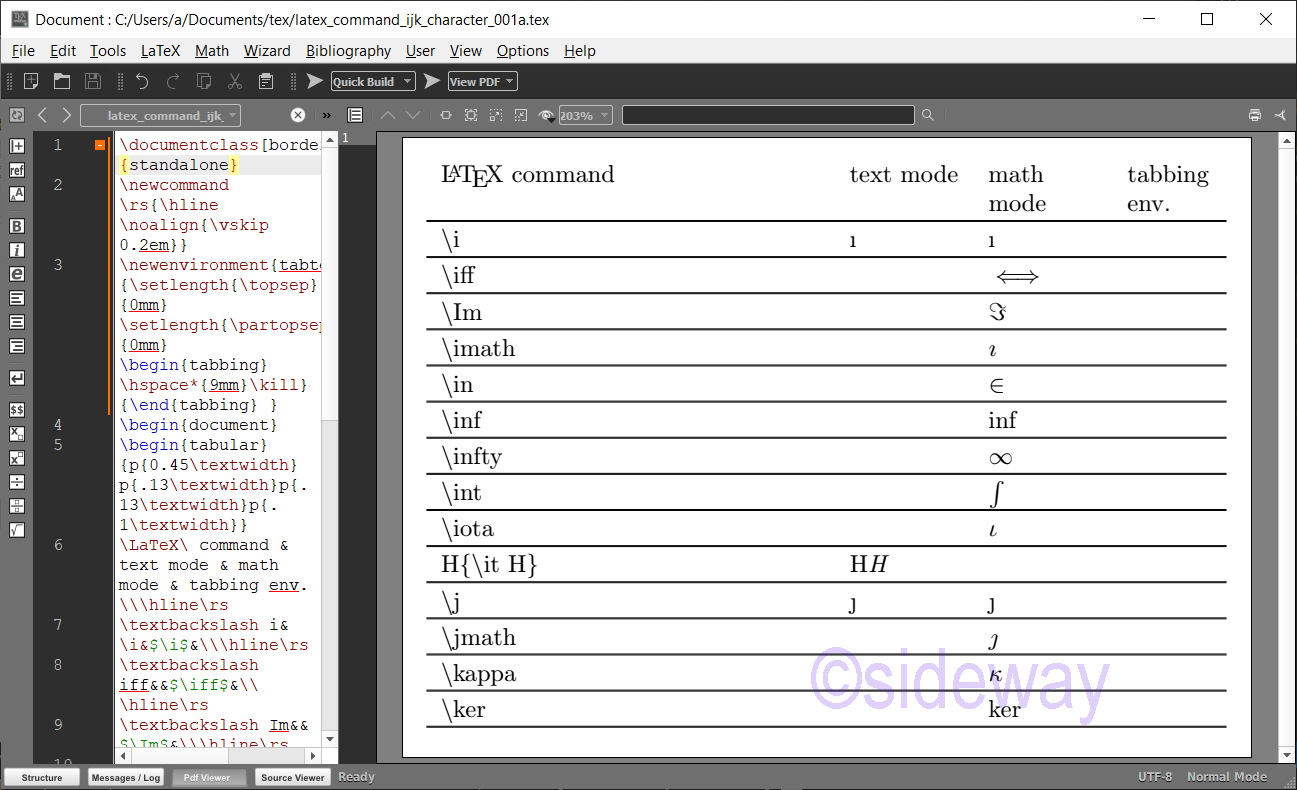output.to from Sideway
Draft for Information Only

# Content

LaTeX \i⋯, \j⋯, \k⋯ Commands for Printing Characters \i⋯, \j⋯ and \k⋯ Commands \i⋯ Command, \j⋯ Command, \k⋯ Command Examples Source and Reference

# LaTeX \i⋯, \j⋯, \k⋯ Commands for Printing Characters

LaTeX uses backslash+i⋯, backslash+j⋯, and backslash+k⋯ combination to print characters.

## \i⋯, \j⋯ and \k⋯ Commands

The typical \i⋯, \j⋯ and \k⋯ Commands for Printing Characters are LaTeX CommandsRemarksMode \iprints a Latin small letter dotless I, ı. \iffprints a long left right double arrow, ⟺.math mode \Imprints a mathematical Fraktur capital J, 𝔍.math mode \imathprints a mathematical italic small dotless I, 𝚤.math mode \inprints a element of, ∈.math mode \infprints math, inf.math mode \inftyprints an infinity, ∞.math mode \intprints an integral, ∫.math mode \iotaprints a Greek small letter iota, ι.math mode \itprints italic type of characters. \jprints a Latin small letter dotless J, ȷ. \jmathprints a mathematical italic small dotless J, 𝚥.math mode \kappaprints a Greek small letter kappa, κ.math mode \kerprints math, ker.math mode

## \i⋯ Command, \j⋯ Command, \k⋯ Command Examples

Examples of LaTeX commands
###### Code
\documentclass[border=10pt,varwidth]{standalone}
\newcommand\rs{\hline\noalign{\vskip 0.2em}}
\newenvironment{tabtest} {\setlength{\topsep}{0mm}\setlength{\partopsep}{0mm}\begin{tabbing}\hspace*{9mm}\kill}{\end{tabbing} }
\begin{document}
\begin{tabular}{p{0.45\textwidth}p{.13\textwidth}p{.13\textwidth}p{.1\textwidth}}
\LaTeX\ command & text mode & math mode & tabbing env.\\\hline\rs
\textbackslash i&\i&$\i$&\\\hline\rs
\textbackslash iff&&$\iff$&\\\hline\rs
\textbackslash Im&&$\Im$&\\\hline\rs
\textbackslash imath&&$\imath$&\\\hline\rs
\textbackslash in&&$\in$&\\\hline\rs
\textbackslash inf&&$\inf$&\\\hline\rs
\textbackslash infty&&$\infty$&\\\hline\rs
\textbackslash int&&$\int$&\\\hline\rs
\textbackslash iota&&$\iota$&\\\hline\rs
H\{\textbackslash it H\}&H{\it H}&\\\hline\rs
\textbackslash j&\j&$\j$&\\\hline\rs
\textbackslash jmath&&$\jmath$&\\\hline\rs
\textbackslash kappa&&$\kappa$&\\\hline\rs
\textbackslash ker&&$\ker$&\\\hline\rs
\end{tabular}
\end{document}
###### Output## Source and Reference

©sideway

ID: 210200025 Last Updated: 2/25/2021 Revision: 0Home 5

Business

Management

HBR 3

Information

Recreation

Culture

Chinese 1097

English 337

Computer

Hardware 153

Software

Application 204

Latex 34

Manim 171

Numeric 19

Programming

Web 285

Unicode 504

HTML 65

CSS 59

ASP.NET 194

OS 409

Python 56

Knowledge

Mathematics

Algebra 30

Geometry 21

Calculus 67

Engineering

Mechanical

Rigid Bodies

Statics 92

Dynamics 37

Control

Natural Sciences

Electric 27

Copyright © 2000-2021 Sideway . All rights reserved Disclaimers last modified on 06 September 2019